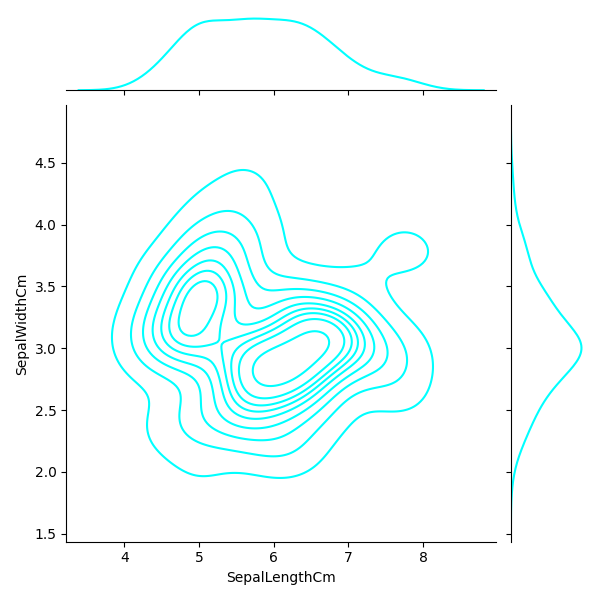﻿ Python Machine learning Scikit-learn: Create a joinplot using “kde” to describe individual distributions on the same plot between Sepal length and Sepal width - w3resource# Python Scikit-learn: Create a joinplot using “kde” to describe individual distributions on the same plot between Sepal length and Sepal width

## Python Machine learning Iris Visualization: Exercise-9 with Solution

Write a Python program to create a joinplot using “kde” to describe individual distributions on the same plot between Sepal length and Sepal width.

Note: The kernel density estimation (kde) procedure visualize a bivariate distribution. In seaborn, this kind of plot is shown with a contour plot and is available as a style in jointplot().

Sample Solution:

Python Code:

``````import matplotlib.pyplot as plt
import pandas as pd
import seaborn as sns
fig=sns.jointplot(x='SepalLengthCm', y='SepalWidthCm', kind="kde", color='cyan', data=iris)
plt.show()
```
```

Sample Output:

``````

Python Code Editor:

Have another way to solve this solution? Contribute your code (and comments) through Disqus.

What is the difficulty level of this exercise?

﻿